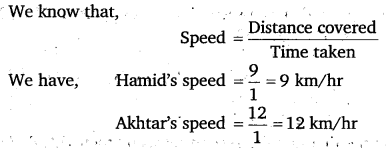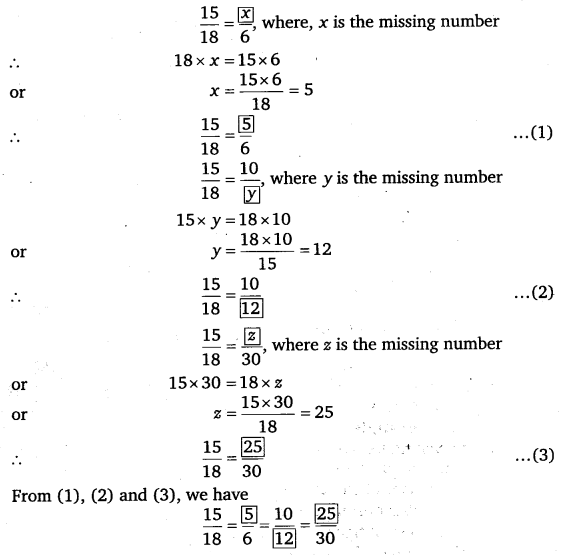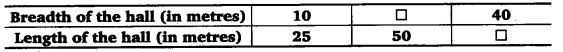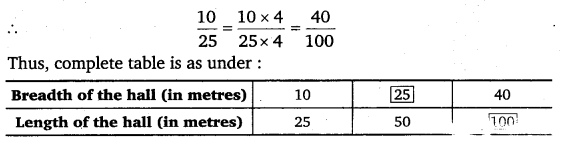# Class 6 Maths NCERT Solutions for Chapter 12 Ratio and Proportion Ex – 12.1

## Ratio and Proportion

Question 1.
There are 20 girls and 15 boys in a class,

(a) What is the ratio of number of girls to the number of boys?
(b) What is the ratio of number of girls to the total number of students in the class?

Solution:
We have,
Number of girls = 20
Number of boys =15
Number of students in the class = (20 + 15) = 35.
(a) Ratio of number of girls to the number of boys
=20 : 15 = 4 : 3
[Dividing the first and second term by their H.C.F. = 5]

(b) Ratio of number of girls to the total number of students
= 20 : 35
= 4 : 7
[Dividing the first and second term by their H.C.F = 5]

Question 2.
Out of 30 students in a class, 6 like football, 12 like cricket and remaining like tennis. Find the ratio of:

(a) Number of students liking football to number of students liking tennis.
(b) Number of students liking cricket to total number of students.

Solution:
We have,
Total number of students = 30
Number of students who like football = 6
Number of students who like cricket = 12
Number of students who like tennis = 30 – (6 +12)
= 30 – 18 = 12
(a) Ratio of number of students liking football to the number of students liking tennis
= 6 : 12 = 1 : 2
[Dividing the first and second term by their H.C.F. = 6]

(b) Ratio of number of students liking cricket to total number of students
= 12 : 30
= 2 : 5
[Dividing the first and second term by their H.C.F. = 6]

Question 3.
See the figure and find the ratio of:

(a)
Number of triangles to the number of circles inside the rectangle.
(b) Number of square to all the figures inside the rectangle.
(c) Number of circles to all the figures inside the rectangle.

Solution:
From the given figure, we have
Number of triangle =3
Number of circles =2
Number of square =2
Total number of figures = 3+ 2 + 2 = 7
(a) Ratio of the number of triangles to the number of circles = 3 : 2
(b) Ratio of the number of squares to all the figures =2 : 7
(c) Ratio of the number of .circles it) all the figures = 2 : 7

Question 4.
Distance travelled by Hamid and Akhtar in an hour are 9 km and 12 km. Find the ratio of speed of Hamid to the speed of Akhtar.

Solution:
We know that,∴ Ratio of the speed of Hamid to the speed of Akhtar
= 9 : 12
= 3 : 4
[Dividing the first term and the second term by their H.C.F. = 3

Question 5.
Fill in the following blanks:Solution:These are equivalent fractions.

Question 6.
Find the ratio of the following:

(a) 81 to 108
(b) 98 to 63
(c) 33 km to 121 km
(d) 30 minutes to 45 minutes.

Solution:
(a) 81 to 108 = 81 : 108 = 3 : 4
[Dividing the first and second terms by their H.C.F. = 27]
(b) 98 to 63 = 98 : 63
= 14 : 9
[Dividing the first and the second terms by their H.C.F-= 7]
(c) 33 km to 121 km = 33 : 121
= 3 : 11
[Dividing the first and the second terms by their H.C.F. =11]
(d) 30 minutes to 45 minutes = 30 : 45
= 2:3
[Dividing the first and the second terms by their H.C.F. = 15]

Question 7.
Find the ratio of the following:

(a) 30 minutes to 1.5 hours
(b) 40 cm to 1.5 m
(c) 55 paise to ₹1
(d) 500 ml to 2 litres

Solution:
(a) 30 minutes to 1.5 hours = 30 minutes: 1.5 x 60 minutes
[∵ 1 hour = 60 minutes]
= 30 min : 90 min = 30 :90 = 1:3
[Dividing the first and the second terms by their H.C.F = 30]

(b) 40 cm to 1.5 m = 40 cm : 1.5 x 100 cm [∵ 1 m = 100 cm]
= 40 cm : 150 cm
= 40 :150 = 4:15
[Dividing the first and the second terms by their H.C.F. = 10]

(c) 55 paise to ₹ 1 = 55 paise : 100 paise [∵ ₹ 1 =100 paise]
= 55 : 100
= 11 : 20
[Dividing the first and the second terms by their H.C.F = 5]

(d) 500 ml to 2 litres = 500 ml: 2 x 1000 ml [∵ 1 litre = 1000 ml]
= 500 : 2000
= 1 : 4
[Dividing the first term and the second terms by their H.C.F = 500]

Question 8.
In a year, Seema earns ? 1,50,000 and saves ? 50,000. Find the ratio of:

(a) Money that Seema earns to the money she saves.
(b) Money that she saves to the money she spends.

Solution:
Seema’s income = ₹150000
Seema’s savings = ₹ 50000
Seema’s expenditure = ₹ 150000 – ₹ 50000
= ₹ 100000
(a) Ratio of Seema’s earnings to her savings
= ₹ 150000 : ₹ 50000
= 150000 : 50000
= 3 : 1
[Dividing the first term and the second term by their H.C.F = 50000]

(b) Ratio of Seema’s savings to her expenditure
= ₹ 50000 : ₹ 100000
= 50000 : 100000 = 1 : 2
[Dividing the first term and the second term by their H.C.F. = 50000]

Question 9.
There are 102 teachers in a school of 3300 students. Find the ratio of the number of teachers to the number of students.

Solution:
We have,
Number of teachers =102
Number of students = 3300
∴ Ratio of the number of teachers to the number of students
= 102 : 3300
= 17 : 550
[Dividing the first term and the second term by their H.C.F = 6]

Question 10.
In a college out of 4320 students, 2300 are girls. Find the ratio of:

(a) Number of girls to the total number of students.
(b) Number of boys to the number of girls.
(c) Number of boys to the total number of students.

Solution:
We have,
Total number of students = 4320
Number of girls = 2300
∴ Number of boys = (4320 – 2300) = 2020
(a) Ratio of number of girls to the total number of students
= 2300 : 4320
= 115 : 216
[Dividing the first term and the second term by their H.C.F = 20]

(b) Ratio of number of boys to number of girls
= 2020 : 2300
= 101 : 115
[Dividing the first term and the second term by their H.C.F = 20]

(c) Ratio of number of boys to the total number of students
= 2020 : 4320
= 101 : 216
[Dividing the first term and the second term by their H.C.F. = 20]

Question 11.
Out of 1800 students in a school, 750 opted basketball, 800 opted cricket and remaining opted table tennis. If a student can opt only one game, find the ratio of:

(a) Number of students who opted basketball to the number of students who opted table tennis.
(b) Number of students who opted cricket to the number of students opting basketball.
(c) Number of students who opted basketball to the total number of students.

Solution:
We have,
Total number of students = 1800
Number of students who opted basketball = 750
Number of students who opted cricket = 800
∴ Number of students who opted table tennis
= 1800 – (750 + 800)
= 1800 – 1550 = 250
(a) Ratio of number of students opting basketball to number of students opting table tennis
= 750 : 250
= 3 : 1
[Dividing the first term and the second term by their H.C.F. = 250]

(b) Ratio of number of students opting cricket to number of students opting basketball
= 800 : 750
= 16 : 15
[Dividing the first term and the second term by their H.C.F. = 50]

(c) Ratio of number of students opting basketball to the total number of students
= 750 : 1800
= 5 : 12
[Dividing the first term and the second term by their H.C.F = 150]

Question 12.
Cost of a dozen pens is ? 180 and cost of 8 ball pens is ? 56. Find the ratio of the cost of a pen to the cost of a ball pen.
Solution:

In order to compare the cost of pen with that of ball pen, we must first find the cost of the same quantity of each of them.
Let us find the cost of one item of each.
Cost of 1 dozen i.e., 12 pens
= ₹ 180
∴ Cost of 1 pen = ₹ 180 -12 = ₹ 15
Cost of 8 ball pens = ₹ 56
∴ Cost of 1 ball pen = ₹ 56 – 8 = ₹ 7
∴ Ratio of the cost of a pen to the cost of a ball pen
= Cost of 1 pen : Cost of 1 ball pen
= ₹ 15 : ₹ 7 = 15 : 7.

Question 13.
Consider the statement: Ratio of breadth and length of a hall is 2:5.

Complete the following table that shows some possible breadths and lengths of the hall.Solution:Question 14.
Divide 20 pens between Sheela and Sangeeta in the ratio of 3:2.

Solution:
Sum of the terms of the ratio = (3 + 2) = 5
Total number of pens = 20Question 15.
Mother wants to divide ? 36 between her daughters Shreya and Bhoomika in the ratio of their ages. If age of Shreya is 15 years and age of Bhoomika is 12 years, find how much Shreya and Bhoomika will get.

Solution:
Shreya’s age : Bhoomika’s age =15 : 12
= 5 : 4
[Dividing the 1st and the 2nd term by their H.C.F = 3]
Since mother wants to divide ₹ 36 between Shreya and Bhoomika in the ratio of their ages. So, the money will be divided in the ratio 5 : 4.
Sum of the terms of the ratio = (5 + 4) = 9Question 16.
Present age of father is 42 years and that of his son is 14 years. Find the ratio of:

(a) Present age of father to the present age of son.
(b) Age of the father to the age of son, when son was 12 years old.
(c) Age of father after 10 years to the age of son after 10 years. Age of father to the age of son when father was 30 years old.

Solution:
We have,
Present age of father = 42 years
Present age pf son = 14 years.

(a) Ratio of present age of father to the present age of son
= 42 years : 14 years
= 42 : 14
= 3 : 1
[Dividing the first term and the second terms by their H.C.F. = 14]

(b) When son’s age is 12 years (i.e., 2 years ago). Then, father’s age
= (42 – 2) = 40 years.
Required ratio = 40 years : 12 years = 40 :12
= 10 : 3
[Dividing the first term and the second terms by their H.C.F = 4]

(c) After 10 years:
Father’s age = (42+10) years = 52 years
Son’s age = (14 +10) years = 24 years
∴ Required ratio = 52 years : 24 years
= 52 : 24
= 13 : 6
[Dividing the first term and the second terms by their H.C.F = 4]

(d) When father’s age is 30 years (i.e., 12 years age), then son’s age
= (14 -12) years = 2 years.
∴ Required ratio = 30 years : 2 years
= 30 : 2
= 15 : 1
[Dividing the first term and the second terms by their H.C.F = 2]